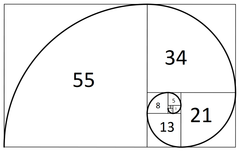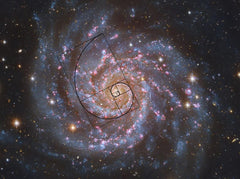# Episode 1: The Fibonacci sequence

In mathematics, the Fibonacci numbers, commonly denoted Fn, form a sequence, the Fibonacci sequence, in which each number is the sum of the two preceding ones. Starting from 1, the next few values in the sequence are:

1, 1, 2, 3, 5, 8, 13, 21, 34, 55, 89, 144, 233, 377, 610, 987…The Fibonacci sequence is one of the simplest and earliest known sequences defined by a recurrence relation, and specifically by a linear difference equation. You may have seen it represented in this diagram form:

The ratio between the numbers in the Fibonacci sequence (1.618033988...) is frequently called the golden ratio or Golden Number, which we will cover in another Episode of our "Nature is All About Math" Series.

The Fibonacci sequence applies to numerous fields, such as Mathematics (the sums of "shallow" diagonals in Pascal's triangle), Computer science (A one-dimensional optimization method, called the Fibonacci search technique, uses Fibonacci numbers), Optics, Financial Market Trading, and you will see it often expressed in Nature:

The Fibonacci sequence appears in the smallest to the largest objects in Nature: Fish ovaries, the flowering of artichoke, the seeds of sunflowers, to storms and galaxies.Above is a microscopic view of the spiral-shaped ovary of an anglerfish in cross-section.Above is a close-up picture of Roman Cauliflower. Its spiral follows the Fibonacci sequence.Most of the time, seeds come from the center of a flower head. Sunflowers are a perfect example of the spiraling patterns.When cut opened, nautilus shells form a logarithmic spiral, composed of chambered sections called camerae. Each new chamber is equal to the size of the two camerae before it (same principle as the Fibonacci sequence), which creates the logarithmic spiral. This proportional growth occurs because the nautilus grows at a constant rate throughout its life until reaching its full size.

And as mentioned earlier, the Fibonacci sequence can be observed at a much larger scale, in Spiral Galaxies:Spiral Galaxies is another example of where Fibonacci’s sequence is apparent. The Milky Way has several spiraled arms that follow in the Fibonacci sequence

The Universe is magnificent and complex, where the relationship between seemingly unrelated things can be found. In Nature, the countless repetitions of patterns that give rise to these fascinating scenarios can often simply be explained mathematically.

If you have just 6 minutes, watch the Magic of Fibonacci numbers, a TED Talk by Arthur Benjamin:

And here is a short video from Cristobal Vila illustrating "The Golden Ratio and Fibonacci Numbers":